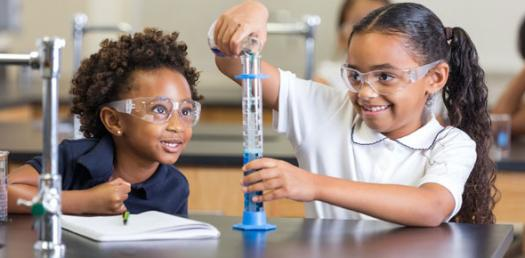# 5th Grade Science Test -matter, Atoms, Force, Motion & Energy

58 Questions | Attempts: 2498
ShareSettingsI created this quiz to test my son's knowledge of 5th grade Science Concepts, or what he should know by the 5th grade!

• 1.
What subatomic particles make up an atom?
• A.

Elements and molecules

• B.

Protons and electrons

• C.

Nucleus and nuetrons

• D.

Electrons,nuetrons and protons

• E.

Particles and molecules

• 2.
The basic unit of structure and function of all living things is a ______________?
• 3.
The measure of the pull of gravity on an object is called it's___________?
• 4.
Characteristics of a substance that can be observed or measured without changing the substance into something else are.....
• A.

Mass

• B.

Matter

• C.

Chemical reactions

• D.

Physical Properties

• E.

Solubility

• 5.
Matter is....?
• A.

How heavy an object is in space.

• B.

Invisible.

• C.

Anything that can be measured.

• D.

So small you can not see it without a microscope.

• E.

Anything that has mass and takes up space.

• 6.
Density is......
• A.

The amount of dense in an object.

• B.

The amount of space an object takes up.

• C.

The concentration of matter in an object.

• D.

The same as weight, unless you are not on earth.

• E.

Is the speed at which light can travel through space.

• 7.
Solubility is the ability of a substance to be.....?
• A.

Measured.

• B.

Weighed.

• C.

Displaced.

• D.

Solid.

• E.

Dissolved.

• 8.
An example of a physical property is.......
• A.

Red

• B.

Small

• C.

Heavy

• D.

Soft

• E.

All of the above

• 9.
What process is the opposite of evaporation?
• A.

Sublimination

• B.

Precipitation

• C.

Condensation

• D.

Boiling point

• E.

Air pressure

• 10.
What state of matter does not have a definitive shape or volume?
• 11.
At what temperature does water boil in F and C?
• A.

98 F and 132 C

• B.

212 F and 100 C

• C.

100 F and 212 C

• D.

110 F and 32 C

• E.

32 F and 0 C

• 12.
Chemical reactions occur when.......
• A.

You mix together any two different substances.

• B.

You combine a powder and a liquid.

• C.

Changes in which one or more new substances are formed.

• D.

Gas is created from a liquid.

• E.

You mix a gas and a liquid to create a solid.

• 13.
Is matter created or destroyed during a physical or chemical change?
• A.

Created

• B.

Destroyed

• C.

Created and Destroyed immediately after.

• D.

Neither Created nor Destroyed.

• E.

It depends on the scientific experiment and which substances you are using.

• 14.
The very tiny center of an atom is called a.....
• A.

Molecule

• B.

Particle

• C.

Subatomic particle

• D.

Nuetron

• E.

Nucleus

• 15.
John Dalton's theory that all matter is made up of tiny particles is called.......?
• A.

The theory of evolution

• B.

The theory of relativity

• C.

The atomic theory of matter

• D.

The atom theory

• E.

The measurement of matter theory

• 16.
Which subatomic particle has a negative charge?
• A.

Electron

• B.

Nuetron

• C.

Proton

• D.

Nucleus

• E.

The theory of negativity.

• 17.
An element is a substance that is made up of only one kind of........
• A.

Nucleus

• B.

Particle

• C.

Matter

• D.

Atom

• E.

Metal

• 18.
What is a molecule?
• A.

Two or more atoms linked together.

• B.

Particles of atoms

• C.

The center of an atom.

• D.

Particles of gas

• E.

A mole who is very "cule"

• 19.
What is the smallest unit of an element that has all the properties of that element?
• A.

A molecule

• B.

An atom

• C.

Nucleus

• D.

An orbital

• E.

A property

• 20.
What is the chemical symbol of  Hydrogen?
• A.

He

• B.

Hy

• C.

H

• D.

Hd

• E.

Hn

• 21.
What is the chemical symbol of Oxygen?
• A.

Ox

• B.

Oh

• C.

Oy

• D.

O

• E.

Oe

• 22.
What is the chemical symbol for Copper?
• A.

Co

• B.

C

• C.

Cu

• D.

Cp

• E.

Ce

• 23.
A compound is a.......
• A.

A mixture

• B.

Substance made of the atoms of two or more elements

• C.

A chemical reaction between two solids

• D.

A two part scientific experiment

• E.

A physical property

• 24.
Any push or pull that causes an object to move, stop or change direction is called?
• A.

Gravity

• B.

Friction

• C.

Energy

• D.

Magnetism

• E.

Force

• 25.
Friction is .......
• A.

The force between two magnets or poles

• B.

A force that opposes, or acts against, motion when two surfaces rub against each other.

• C.

When an object is attracted to another object.

• D.

Heat

• E.

The speed of magnetic force.

## Related TopicsBack to top
×

Wait!
Here's an interesting quiz for you.